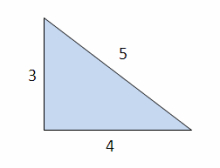# Sides in ratio

The sides of the triangle are in a ratio of 2: 8: 5. Find the dimensions of the remaining sides if the longest side is 32 cm.

cm
cmDid you find an error or inaccuracy? Feel free to write us. Thank you!Tips to related online calculators
Need help to calculate sum, simplify or multiply fractions? Try our fraction calculator.
Check out our ratio calculator.